##### Math For Real Life For Dummies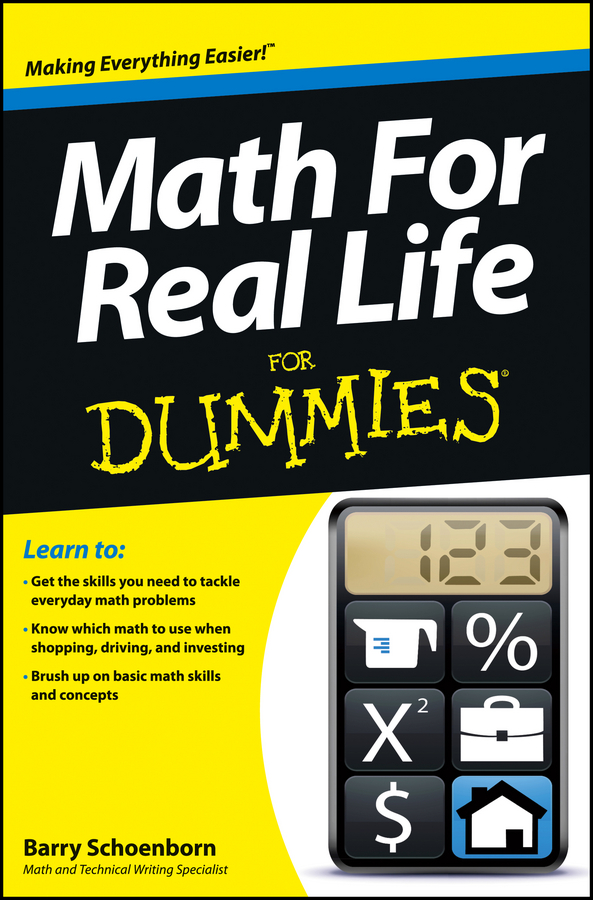To multiply numbers, you need to know how to work with the different times signs as well as parentheses. If you multiply large numbers, you also need to know how to stack them in columns, so that you can work with them.

Multiplication is often described as a sort of shorthand for repeated addition. For example, when you multiply 4 by 3, you are adding 4 to itself 3 times: 4 + 4 + 4 = 12.

Similarly:

9 6 means add 9 to itself 6 times: 9 + 9 + 9 + 9 + 9 + 9 = 54

100 2 means add 100 to itself 2 times: 100 + 100 = 200

Although multiplication isn’t as warm and fuzzy as addition, it’s a great timesaver. For example, suppose you coach a Little League baseball team and you’ve just won a game against the toughest team in the league. As a reward, you promised to buy three hot dogs for each of the nine players on the team. To find out how many hot dogs you need, you could add 3 together 9 times. Or you can save time by multiplying 3 times 9, which gives you 27. Therefore, you need 27 hot dogs (plus a whole lot of mustard and sauerkraut).

When you multiply two numbers, the two numbers that you’re multiplying are called factors, and the result is the product.

In multiplication, the first number is also called the multiplicand and the second number is the multiplier. But almost nobody ever remembers these words.

## Use signs and parentheses in multiplication

When you’re first introduced to multiplication, you use the times sign (). However, algebra uses the letter x a lot, which looks similar to the times sign, so people often choose to use other multiplication symbols for clarity.

Keep in mind that in math beyond arithmetic, the symbol · replaces .

For example,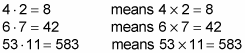That’s all there is to it. Just use the symbol anywhere you would’ve used the standard times sign ().

Remember that in math beyond arithmetic, using parentheses without another operator stands for multiplication. The parentheses can enclose the first number, the second number, or both numbers. For example,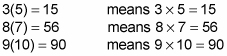However, notice that when you place another operator between a number and a parenthesis, that operator takes over. For example,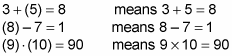Note: In the third example, you don’t really need the , but it isn’t doing any harm, either.

## Multiply larger numbers

The main reason to know the multiplication table is so you can more easily multiply larger numbers. For example, suppose you want to multiply 53 7. Start by stacking these numbers one on top of another with a line underneath, and then multiply 3 by 7. Because 3 7 = 21, write down the 1 and carry the 2: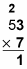Next, multiply 7 by 5. This time, 5 7 is 35. But you also need to add the 2 that you carried over, which makes the result 37. Because 5 and 7 are the last numbers to multiply, you don’t have to carry, so write down the 37 — you find that 53 7 = 371: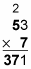When multiplying larger numbers, the idea is similar. For example, suppose you want to multiply 53 by 47. (The first few steps — multiplying by the 7 in 47 — are the same, so you pick up with the next step.) Now you’re ready to multiply by the 4 in 47. But remember that this 4 is in the tens column, so it really means 40. So to begin, put a 0 directly under the 1 in 371: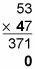This 0 acts as a placeholder so that this row is arranged properly.

When multiplying by larger numbers with two digits or more, use one placeholding zero when multiplying by the tens digit, two placeholding zeros when multiplying the hundreds digit, three zeros when multiplying by the thousands digit, and so forth.

Now you multiply 3 4 to get 12, so write down the 2 and carry the 1: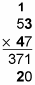Continuing, multiply 5 4 to get 20 and then add the 1 that you carried over, giving a result of 21: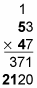To finish up, add the two products (the multiplication results):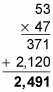So 53 47 = 2,491.